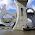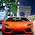## Monday, September 14, 2009

### Higher Order Functions in Java

In order to understand future examples, we first have to discuss how to use higher-order functions in Java, and how to write anonymous inner classes. This post will have nothing to do with multi-stage programming.

Let's write a program that can print out data tables for different mathematical functions. For example, for a function that multiplies by two, f(x) = 2x, we want to print something like this:

`x                      f(x)       -5.0000000000       -10.0000000000       -4.0000000000        -8.0000000000       -3.0000000000        -6.0000000000       -2.0000000000        -4.0000000000       -1.0000000000        -2.0000000000        0.0000000000         0.0000000000        1.0000000000         2.0000000000        2.0000000000         4.0000000000        3.0000000000         6.0000000000        4.0000000000         8.0000000000        5.0000000000        10.0000000000`

We can write a function like this:

`    public static void printTableTimesTwo(double x1,                                          double x2,                                          int n) {        assert n>1;        double x = x1;        double delta = (x2-x1)/(double)(n-1);        System.out.println("x                      f(x)");        System.out.printf("%20.10f %20.10f\n", x, x*2);        for(int i=0; i<(n-1); ++i) {            x += delta;            System.out.printf("%20.10f %20.10f\n", x, x*2);        }    }`

The parameter `x1` determines the lower end of the interval, `x2` the upper end, and `n` determines how many values should be printed. `n` needs to be at least `2` to print out the values at `x1` and `x2`. We can generate the table above with this call:

`        printTableTimesTwo(-5, 5, 11);`

What if we want to print out the values of a different function, for example f(x) = x + 4? We can write a new function:

`    public static void printTablePlusFour(double x1,                                          double x2,                                          int n) {        assert n>1;        double x = x1;        double delta = (x2-x1)/(double)(n-1);        System.out.println("x                      f(x)");        System.out.printf("%20.10f %20.10f\n", x, x+4);        for(int i=0; i<(n-1); ++i) {            x += delta;            System.out.printf("%20.10f %20.10f\n", x, x+4);        }    }`

This involves a lot of code duplication, though. The only parts that actually differ are the two occurrences of `x*2` and `x+4`. How can we factor that difference out?

Let's write an interface that we can use for any kind of function that takes in one parameter and returns one parameter f(x) = y is an example of such a function.

`public interface ILambda<R,P> {    public R apply(P param);}`

This interface is called `ILambda` and it has one method, `apply`. We used Java generics and didn't specify the return type and the type of the parameter; instead, we just called them `R` and `P`, respectively. A function that takes in a `Double` and that returns a `Double`, like f(x) = y, can be expressed using a `ILambda<Double,Double>`. A function taking a `String` and returning an `Integer` would use `ILambda<String,Integer>`.

Now we can write our f(x) = 2x and f(x) = x + 4 functions using `ILambda`:

`    public class TimesTwo implements ILambda<Double,Double> {        public Double apply(Double param) { return param*2; }    }    public class PlusFour implements ILambda<Double,Double> {        public Double apply(Double param) { return param+4; }    }`

Now we can write one `printTable` method that takes in an `ILambda<Double,Double>` called `f` representing the function, in addition to the parameters `x1`, `x2` and `n`, as before:

`    public static void printTable(ILambda<Double,Double> f,                                  double x1,                                  double x2,                                  int n) {        assert n>1;        double x = x1;        double delta = (x2-x1)/(double)(n-1);        // f.apply(x) just means what f(x) means in math!        double y = f.apply(x);        System.out.println("x                      f(x)");        System.out.printf("%20.10f %20.10f\n", x, y);        for(int i=0; i<(n-1); ++i) {            x += delta;            y = f.apply(x);            System.out.printf("%20.10f %20.10f\n", x, y);        }    }`

Note that when we want to print out the y-value, we just write `f.apply(x)`, which looks very similar to f(x) in mathematics. It means exactly the same.

We can print out the tables for our two functions using:

`        printTable(new TimesTwo(), -5, 5, 11);        printTable(new PlusFour(), -5, 5, 11);`

We have to create new objects for the functions: The first time we call `printTable` we pass a new `TimesTwo` object; the second time, we pass a new `PlusFour` object.

We can now define as many functions as we like without having to rewrite the `printTable` function. For example, we can easily write a square root function and use it very easily:

`    public class SquareRoot implements ILambda<Double,Double> {        public Double apply(Double param) {            return Math.sqrt(param);        }    }// ...        printTable(new SquareRoot(), -5, 5, 11);`

The really neat thing is that we can even define a new function on-the-fly, without having to give it a name. We do that using anonymous inner classes in Java. Here, we call `printTable` and pass it a new object that implements `ILambda<Double,Double>`.

`        printTable(new ILambda<Double,Double>() {            public Double apply(Double param) {                return param*param;            }        }, -5, 5, 11);`

We define a new `ILambda` from `Double` to `Double` without giving it a name. When we use anonymous inner classes, we need to fill in all the methods that are still abstract. Here, it is just the `apply` method.

The method `printTable` is now a "higher order function", because conceptually it is a function that takes another function as input.

Questions:

1. What does the anonymous `ILambda<Double,Double>` in the example above compute? What's the mathematical function it represents?

2. How would you print a table for the function f(x) = x2 + 2x?

1.Wow, thank you. That is excatly what I was looking for.

I really still miss the "true lambdas" in Java which can access members of the enclosing scope.
In java 7 there should be clojures, but our company uses jdk version 5.

2.3.4.5.6.7.8.9.